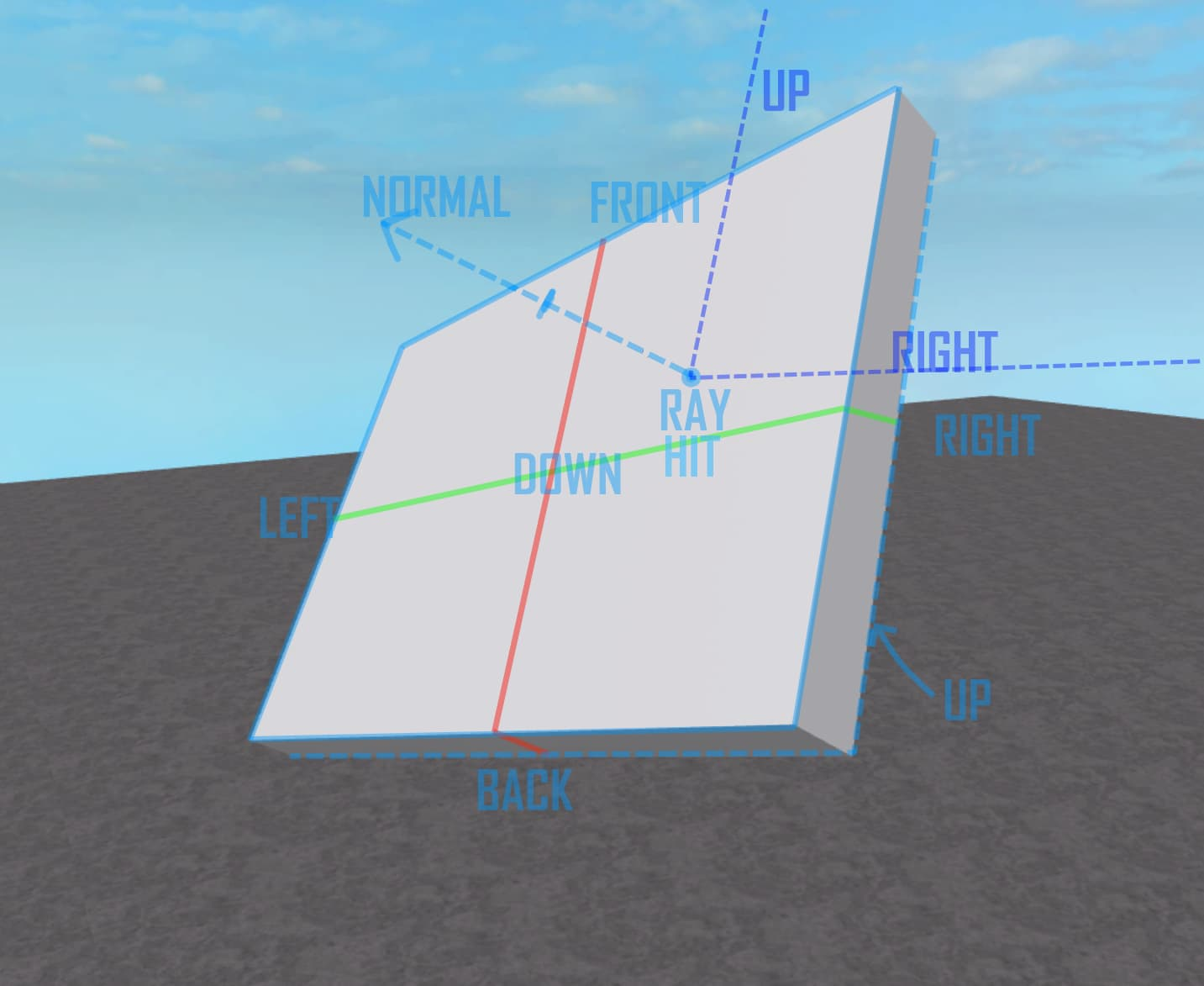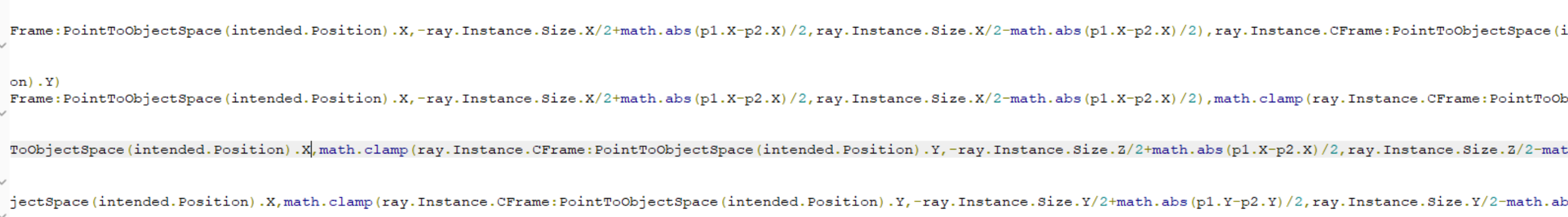# How to Get Size From Surface

So say you have a part that’s flipped sideways, and the top facing normal corresponds to its Z face, but normal vector is ~(0,1,0), so it would get the (X,0,Z) size, but that’s corresponding to the part’s top face, and I want the size of its Z surface, which is (0,1,0).

So yeah it’s just an example and don’t give me some hacky solutions based only around this solution, I just want to know how to get the size of a surface of the part, no matter its orientation.

I have a part that is sized (50,40,0) and is rotated (90,0,0). When I’m hovering over the top side, with normal (0,1,0), I expect the function to return (50,1,40). Instead, what it returns, is still (50,40,0).

In other words, it would be as if to lay one part on top of the face of the other, rotated (0,0,0), and sized (50,40,1), it would encompass the entire surface of the latter part which I am hovering over, yet having the rotation of (0,0,0), whereas the latter part would have the rotation of (90,0,0)

The part in red would be the part which I have given the example that it would encompass the surface my mouse would be hovering on. It has the size (50,1,40) and rotation (0,0,0) and is positioned 1 stud above the part with the decal on it.
The decal on the part with the decal on it is facing its front side, and its size is (50,40,1), and is rotated (90,0,0).

In another visual analogy, I want to find the hypothetical size of this red part with its decal facing top. which in this case, would be (50, 0.001, 40)

2 Likes

Try checking the parts CFrame.upVector and get the size based on that and the normal

I should be able to help better when I’m back at my pc

Okay, you may be on to something here, but I want this to work with whatever surface based on a raycast using your mouse. So for example, if we the raycast normal is (1,0,0), but the part is rotated (0,0,90), then if you got the area using the part’s upvector and lookvector, you would get the area of the surface currently facing top, since the part is rotated (0,0,90).

Adding on, by area I meant like Vector2. I read this other post (the only one that asks about this other than me) but it gives a very inconcise answer and I’m not sure if their solution was right, because the OP never gave any follow up which is just straight up lame

How will we discover the up and right vectors relative to the surface normal? That’s the only information we have, so what if the part looks like this?

Just so you guys know this is what I mean by discovering the up and right vectorsWithout up and right vectors and only the look vector, it’s impossible to determine the size of the area. I need someone with incredibly advanced knowledge of CFrames, Vectors, Geometry, and Roblox… hint hint… Egomoose

I like how every other question this devforum gets answers within like 3 seconds and I have to wait hours and days and even weeks for any sort of remotely close answer

I just found this post from a little bit ago that seems very simular to what you are asking, perhaps you should look some more because googling “roblox how to get area from surface” came up with many results.

That is literally the post I mentioned twice in this thread and if you haven’t realised I posted there three times already

And yes I did google that term along with several other variations and the post you linked is the only post mentioning this, all the other posts on google are irrelevant. If I’m asking this question on devforum that usually means I’ve already googled things.

I just read through again and still don’t see it mentioned anywhere here, I recall you saying there is no posts about this so… idk anything about this topic but you were saying you had no luck so I thought I’d search aroundArea of a square: Width times Length. Area of a cube: Width times Length times Depth. Simple math.

@TehEpikKeith

I read this other post (the only one that asks about this other than me) but it gives a very inconcise answer and I’m not sure if their solution was right, because the OP never gave any follow up which is just straight up lame

@DaRealDevGaming
I’m in high school. You think I wouldn’t know how to calculate the area of a square? I’m trying to find the area of any surface of any part no matter its rotation or size or position.
Take a moment to read what little I have wrote in this thread and maybe you can get a better idea of what I want to achieve.@SatrapRezaPahlavi
Anyways I have a theory
Given that a part’s CFrame has an UpVector, LookVector and RightVector, you can compare the angle between those vectors and the normal vector of a raycast (ray.Normal)

So, steps:

1. Create a table mapping out the values and the results we need
``````-- assuming you did raycast above this code --

if (hitNormal) then
local dotY = hitNormal:Dot(Vector3.new(0,1,0))
local dotX = hitNormal:Dot(Vector3.new(1,0,0))
local dotZ = hitNormal:Dot(Vector3.new(0,0,1))
if (math.abs(dotY) >= 0.9) then
if (dotY < 0) then
surface = Enum.NormalId.Bottom
else
surface = Enum.NormalId.Top
end
elseif (math.abs(dotX) >= 0.9) then
if (dotX < 0) then
surface = Enum.NormalId.Left
else
surface = Enum.NormalId.Right
end
else
if (dotZ < 0) then
surface = Enum.NormalId.Front
else
surface = Enum.NormalId.Back
end
end
end
``````

Oh nevermind about that CFrame theory, I found this on another DevForum post. lol

So first work done. Now we map up the formula to calculate area for each side.

``````local s = part.Size
local sideToAreaMapping = {
[Enum.NormalId.Top] = s.X*s.Z,
[Enum.NormalId.Bottom] = s.X*s.Z,
[Enum.NormalId.Left] = s...
``````

Gah I don’t remember which face uses which axis but
left area = right area
front area = back area

So to get the final area

``````local areaOfSideAtNormal = sideToAreaMapping[surface]
``````

This is only accurate for rectangle and squares – You would need different sideToAreaMapping for different shapes

I’ve been reading this thread for the past hour, sorry that I skimmed over you claiming someone just dropped a post randomly when they marked their solution as the answer, which is what you are supposed to do? Maybe you really should touch some grass

There has to be a more elegant solution than just this. I already have the function to find which Enum the face of the normal is.

Okay, good luck
If I were you I’d stick with what works but then again, I’m not the one facing the problem…

What you gave is a hacky solution which uses manmade tables. I want this to be done completely with only mathematical equations. I have to run three if statements each inside of three if statements, which is sad and bad.

Tell me. Can you read this code?
I sure can’t